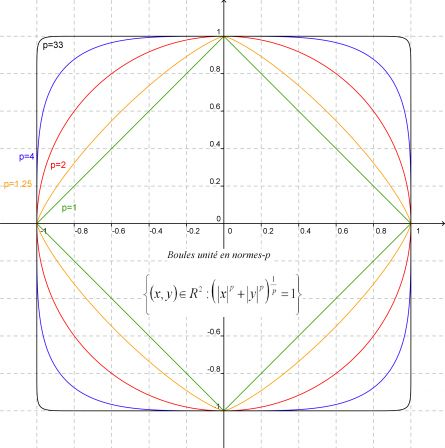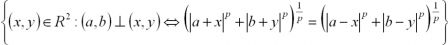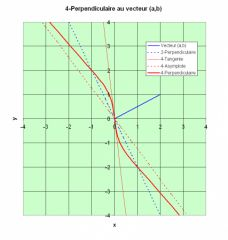# Lucas Borboleta

## Mathématiques

dimanche 1 décembre 2013

## Inner Product Space Characterization by Isometry Group over Unity Sphere

Why “power 2” in the Pythagorean theorem ?

Let us assume a geometry over the set E considered as 1) a vector space over the real field ; 2) with finite dimensions ; 3) equiped with a norm.

Which additional axioms have to be added in order to conclude that E is euclidean, or equivalently, that its norm is deriving from an inner product. Such additionnal axioms are searched in topology and in group formulations, as opposed to calculational ones, like the “polarisation identity”.

The attached document gathers theorems that proof the following axiom solution: 4) if a group G of isometries allows transportation between any pair of points of the unity sphere of E, then E is euclidean.

Borboleta_2013_Inner-Product-Space-Characterization-by-Isometric-Group-over-Unity-Sphere.pdfdimanche 24 novembre 2013

## Return on the 1932 proof of the Auerbach Theorem about Bounded Linear Groups

In 1932, Auerbach proved that, in any finite vector normed space, each bounded linear group left invariant a quadratic and positive form. This work revisits his proof with modern notation, and aims at paying attention to weaknesses: Borboleta_2013_Return-on-Auerbach-Theorem-for-bounded-linear-group.pdf.

dimanche 14 juillet 2013

## MathSAN: Maths with Symbolic Ascii Notation

How to write and work electronic mathematical text without WYSIWYG software or LaTeX?  This memo aims at proposing  MathSAN, an acronym for Mathematics with Symbolic Ascii Notation, focusing on mathematical symbolic or semantic rather than typographic rendering: PDF memo, text memo.

Samples:
```A .i B := {x: x .e A and x .e B }
sum[ i .e NAT ; 1<= i <= n ] = n*(n+1)/2
der[ x**3*y**2 ; x.2 ; y ] = 12*x*y
int[ x**2*dx ; a <= x <= b] = (b**3 - a**3)/3

```

dimanche 14 octobre 2012

## Les Comptes rendus hebdomadaires des séances de l'Académie des sciences (France) sur le web par Gallica BNF

Les Comptes rendus hebdomadaires des séances de l'Académie des sciences (France) sont publiés par la Bibliothèque Nationale de France. Le site web http://gallica.bnf.fr en permet la consultation.

Par exemple, le théorème de 1932 de Mazur et Ulam est lisible des pages 946 à 948 dans le volume référencé http://gallica.bnf.fr/ark:/12148/bpt6k31473

## Return on the 1932 proof of the Mazur-Ulam Theorem

In 1932, Mazur and Ulam proved that any isometry f of a normed space E , with f(0)=0, is necessarily a linear transformation. The following article revisits that theorem and its proof: Borboleta_2012_Return-on-the-1932-proof-of-the-Mazur-Ulam-Theorem.pdf

dimanche 23 septembre 2012

## Homework on Jordan-Neumann Inner Product Space Characterization

Here is an homework that revisits  (in the simplest case of real vector space) the proof of 1935 by Jordan and von Neumann, that a vector normed space satisfying the parallelogram law can be equiped with an inner productBorboleta_2012_Homework-on-Jordan-Neumann-Inner-Product-Spaces-Characterization.pdf.

In this proof, the triangular inequality proprety of the norm is invoked for a continuity argument, but otherwise not used.

samedi 8 septembre 2012

## Comment trouver des travaux de caractérisation de l'espace euclidien parmi les espaces vectoriels normés ?

J'ai appris d'une question sur le site http://mathoverflow.net/ que les mots clés fructueux pour trouver sur le Net des travaux de caractérisation de l'espace euclidien parmi les espaces vectoriels normés sont " Characterization of Inner Product Spaces".

Avec ce sésame, on moissonne des livres et des articles. Il n'y a plus qu'à travailler à les comprendre.

Merci aux contributeurs de MathOverflow.

dimanche 15 juillet 2012

## The group of linear isometries in R2 is discrete and finite group any p-norm, except for p=2!

The euclidean norm is special among the p-norms. Indeed, let us focus on the plan R2. The set of linear isometries in R2, for any p-norm, always composes a group. But for any p-norm, except for p=2, such group is discrete and finite; basiclly it is generated by median and diagonal reflections. Only for p=2, such group of linear isometries is continous. This is showed in the article Borboleta_2012_Linear-Isometries-in-R2-for-P-Norms.

samedi 4 décembre 2010

## Boules unité en normes-p

Voici à quoi ressemble les boules "unité", généralisation du cercle "unité", en normes-p. Le logiciel GeoGebra a permis de les tracer aisément.## Les perpendiculaires de R2 en norme-p ne sont presque jamais droites sauf pour p=2 !

Parmi les normes-p de R2, seule la norme-2 induit des perpendiculaires toujours droites. C'est ce que montre l'article Les-perpendiculaires-en-norme-p-ne-sont-pas-des-droites-sauf-pour-p-egal-2.pdf .C'est un pas de plus dans le cheminement pour la caractérisation de l’espace euclidien, dans un premier temps, par des contre-exemples de normes déconnectées d’un produit scalaire

dimanche 21 novembre 2010

## Les perpendiculaires de R2 en norme-4 ne sont presque jamais droites !

Dans un espace vectoriel fini, toutes les normes sont équivalentes du point de vue topologique.

Sont-elles aussi équivalentes pour l'orthogonalité ?

L'article annexé au billet répond négativement par le contre exemple de la norme-4 dans R2: Les perpendiculaires n'y sont presque jamais droites ! Voir la figure ci-dessous. Ceci souligne une singularité de l'espace euclidien. Lire la suite dans l'article Les-perpendiculaires-de-R2-en-norme-4-ne-sont-presque-jamais-droites.pdf